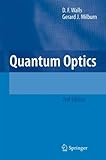# Calculations on Coherent States and Squeezed States (2)Quantum Optics

This is intended to fill some gaps in calculation details in the book above.

#### 2.6 Variance in the Electric Field

HamiltonianElectric field operatorTime evolution (in the Heisenberg picture)

1.2.3.For 1.,, hence.
2. is conjugate of 1. 3. follows directly from 1. and 2.

4.Rewriting 3. withTime dependent variance of E(t)

5.Hence,results in 5.
where6.whenApply the Schroedinger's uncertainty relationEspecially when, under the condition that, you get the next result.

7.Hence, for(unsqueezed coherent state), the variance becomes constant whereas for(squeezed coherent state), the variance flactuates with the angular velocity.

8.This follows from 3. and.

Especially for, it results inThe following shows graphs offor variouss.

#### Schematic view of oscillation

Electric field operatorTime evolutionExpected valueIn terms of, it corresponds to:Define the rotated coordinatesfor some fixed.
Then at,. Hence,These results can be summarized in the following diagram.

#### 3.7.1 Squeezed State

Photon number fluctuation for the squeezed state.

1.2.whereFor 1.,For 2.,sinceHence,sinceAs a result,sinceFrom these results, you can see thatfor(non-squeezed coherent state) since it obeys the Poisson distribution.

On the other hand, when:

for,. It becomes the sub-Poissson.

for,. It becomes the super-Poissson.

Especially, for(Squeezed vacuum)],. It's always the super-Poission.